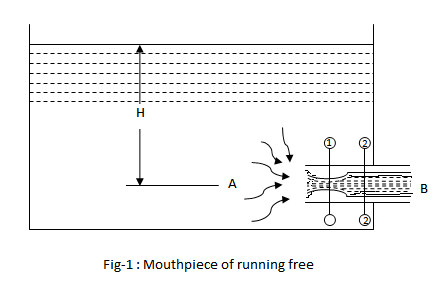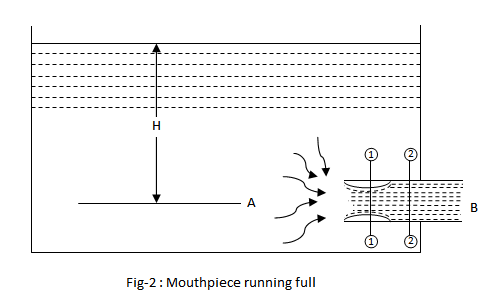•http://facebook.com/
•https://www.google.com/accounts/o8/id
•https://me.yahoo.com

# Internal Mouthpiece

Discharge and Pressure in an Internal Mouthpiece

## Introduction

An internal mouthpiece, extending into the fluid (i.e., inside the vessel) is known as Re-entrant or Borda's mouthpiece. There are mainly two types of internal mouthpieces depending upon their nature of discharge:
• Mouthpiece running free
• Mouthpiece running full

In this page we will discuss about the discharge and pressure in an internal mouthpiece when it runs free and full.

## Discharge Through An Internal Mouthpiece

### Mouthpiece Running Free

If the jet, after contraction, does not touch the sides of the mouthpiece, it is said to be running free as shown in fig-1. It has been experimentally found that if the length of the mouthpiece extending into the fluid is less than 3 times the diameter of the orifice, it will run free.Consider a mouthpiece running free. Let,

• = Height of liquid above the mouthpiece,
• = Area of orifice or mouthpiece,
• = Area of the contracted jet,
• = Velocity of the liquid
• = Specific weight of the liquid

We know that pressure of the liquid on the mouthpiece,

Force acting on the mouthpiece = Pressure Area

Mass of the liquid flowing per second

Momentum of the flowing liquid = Mass Velocity

Since the water is initially at rest, therefore initial momentum = 0

and change of momentum

According to Newton's second Law of Motion, the force is equal to the rate of change of momentum. Therefore equating equations (1) and (3),

Coefficient of contraction, = = 0.5

We know that coefficient of discharge (assuming equal to 1),

Discharge,

### Mouthpiece Running Full

If the jet, after contraction, expands and fills up the whole mouthpiece, it is said to be running full as shown in fig-2. If the length of the mouthpiece is more than 3 times the diameter of the orifice, it will run full.Consider a mouthpiece running full. Let,

• = Height of liquid above the mouthpiece,
• = Area of the flow at the vena contracta,
• = Velocity of the liquid at the outlet
• = Velocity of the liquid at the vena contracta

Since the liquid is flowing continuously, therefore

Previously we have seen that the coefficient of contraction in an internal mouthpiece is 0.5.

Therefore substituting the value of = 0.5 in the above equation,

We see that the jet after passing through section 1-1 suddenly enlarges at section 2-2. Therefore there will be a loss of head due to sudden enlargement. We know that the loss of head due to sudden enlargement,

Applying Bernoulli's equation to points A and B,

Actual Discharge =

We know that theoretical discharge

Coefficient of discharge,Cd = Actual discharge / Theoretical discharge

and discharge,

Example:
[metric]
##### Example - Discharge through an Internal Mouthpiece running free
Problem
A Borda's mouthpiece of 50mm diameter is provided on one side of a tank containing water up to a height of 3m above the center line or the orifice. Find the discharge through the mouthpiece, if the mouthpiece is running free.
Workings
Given,
• = 50 mm = 0.05m
• = 3m

The area of the mouthpiece,

and discharge through the mouthpiece,
Solution
The discharge through the mouthpiece = 7.53 liters/s

## Pressure In An Internal Mouthpiece

Consider a vessel, open to atmosphere at its top, having an orifice with an internal mouthpiece, as shown in fig-3. We know that the jet of the liquid, on entering the mouthpiece, will first contract up to vena contracta C and then expand and fill up the whole orifice as shown in figure.Let,
• = Height of liquid above the mouthpiece
• = Absolute pressure head at vena contracta
• = Coefficient of contraction
• = Velocity of liquid at outlet
• = Velocity of liquid at vena contracta

Applying Bernoulli's equation to point A and C,

From equation (5) we know that,

substituting the value of in equation (7)

This shows that the absolute pressure head at vena contracta is less than the atmospheric pressure by an amount equal to height of the liquid above the vena contracta. A little consideration will show that the absolute pressure head at vena contracta will be zero, when of water

It means that if this condition is reached (i.e., height of water above the mouthpiece reaches 10.3m), the flow of the liquid would be no longer steady. This means that the mouthpiece will not run full at its outlet and the jet will flow straight without touching the walls of the mouthpiece. But, in actual practice, this condition also reaches at 7.5 m.

Example:
[metric]
##### Example - Pressure in an Internal Mouthpiece
Problem
A re-entrant mouthpiece of 75mm diameter is discharging water under a constant head of 3.5m. Determine the absolute pressure head at vena contracta, if the atmosphere pressure head be 10.3 m of water.
Workings
Given,
• = 75 mm = 0.075 m
• = 3.5 m
• = 10.3 m

We know that the absolute pressure head at the vena contracta of an internal mouthpiece.

H_c = H_a - H = 10.3 - 3.5 = 6.8 m
Solution
Absolute pressure head = 6.8 m